## Parametric vs. Nonparametric Approach to Estimations

Parametric statistics assume that the unknown CDF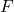belongs to a family of CDFs characterized by a parameter (vector)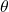. As the form ofis assumed, the target of estimation is its parameters. Thus, all uncertainty aboutis comprised of uncertainty about its parameters. Parameters are estimated by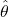, and estimates are be substituted into the assumed distribution to conduct inference for the quantities of interest. If the assumed distributionis incorrect, inference may also be inaccurate, or trends in the data may be missed.

To demonstrate the parametric approach, consider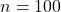independent and identically distributed random variables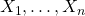generated from an exponential distribution with rate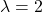. Investigators wish to estimate the 75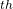percentile and erroneously assume that their data is normally distributed. Thus,is assumed to be the Normal CDF but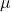and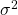are unknown. The parametersand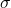are estimated in their typical way by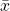and, respectively. Since the normal distribution belongs to the location-scale family, an estimate of the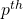percentile is provided by,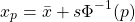where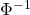is the standard normal quantile function, also known as the probit.

set.seed(12345)
library(tidyverse, quietly = T)

# Generate data from Exp(2)
x <- rexp(n = 100, rate = 2)

# True value of 75th percentile with rate = 2
true <- qexp(p = 0.75, rate = 2)
true

##  0.6931472

# Estimate mu and sigma
xbar <- mean(x)
s    <- sd(x)

# Estimate 75th percentile assuming mu = xbar and sigma = s
param_est <- xbar + s * qnorm(p = 0.75)
param_est

##  0.8792925


The true value of the 75percentile of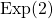is 0.69 while the parametric estimate is 0.88.

Nonparametric statistics make fewer distributions about the unknown distribution, requiring only mild assumptions such as continuity or the existence of specific moments. Instead of estimating parameters of,itself is the target of estimation.is commonly estimated by the empirical cumulative distribution function (ECDF)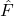,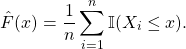Any statistic that can be expressed as a function of the CDF, known as a statistical functional and denoted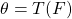, can be estimated by substitutingfor. That is, plug-in estimators can be obtained as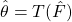.

## Motivation

For observed pairs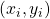,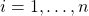, the relationship between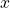and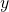can be defined generally as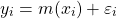where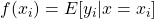and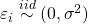. If we are unsure about the form of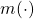, our objective may be to estimatewithout making too many assumptions about its shape. In other words, we aim to “let the data speak for itself”.

Simulated scatterplot of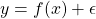. Here,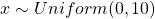and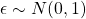. The true function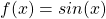is displayed in green.

Non-parametric approaches require only thatbe smooth and continuous. These assumptions are far less restrictive than alternative parametric approaches, thereby increasing the number of potential fits and providing additional flexibility. This makes non-parametric models particularly appealing when prior knowledge about‘s functional form is limited.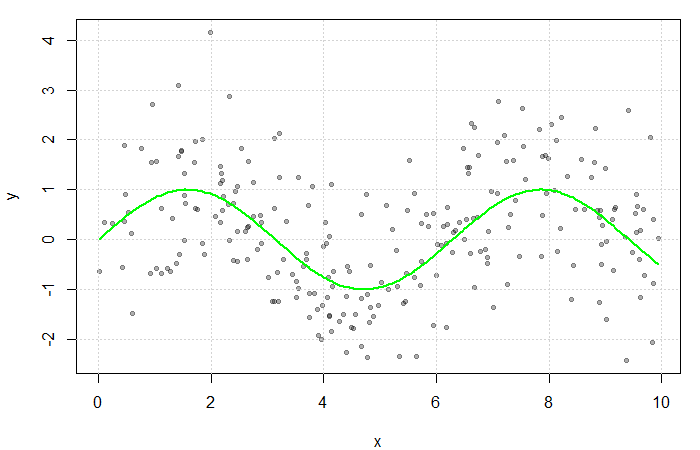## Estimating the Regression Function

If multiple values ofwere observed at each,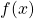could be estimated by averaging the value of the response at each. However, sinceis often continuous, it can take on a wide range of values making this quite rare. Instead, a neighbourhood ofis considered.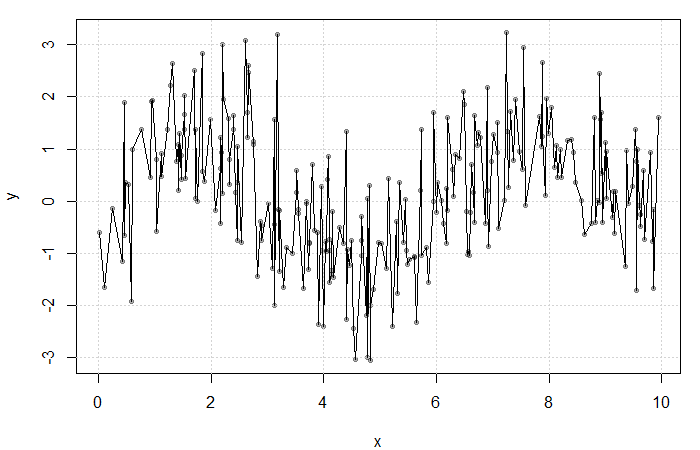Result of averaging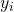at each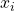. The fit is extremely rough due to gaps inand lowfrequency at each.

Define the neighbourhood aroundas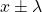for some bandwidth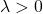. Then, a simple non-parametric estimate of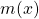can be constructed as average of the‘s corresponding to thewithin this neighbourhood. That is,

(1)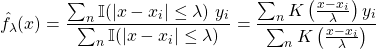where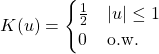is the uniform kernel. This estimator, referred to as the Nadaraya-Watson estimator, can be generalized to any kernel function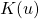(see my previous blog bost). It is, however, convention to use kernel functions of degree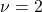(e.g. the Gaussian and Epanechnikov kernels).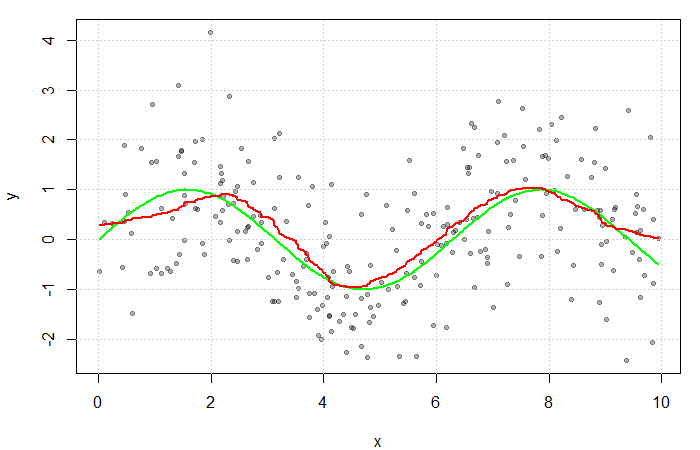The red line is the result of estimatingwith a Gaussian kernel and arbitrarily selected bandwidth of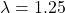. The green line represents the true function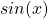.